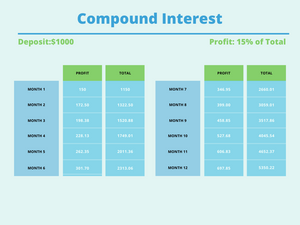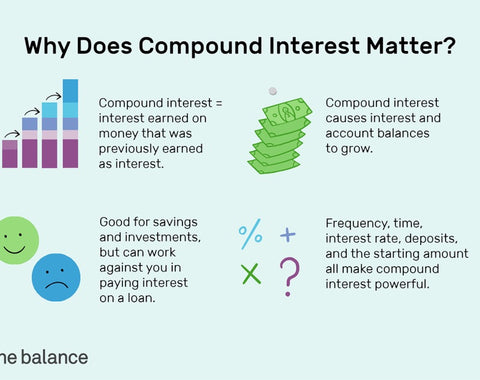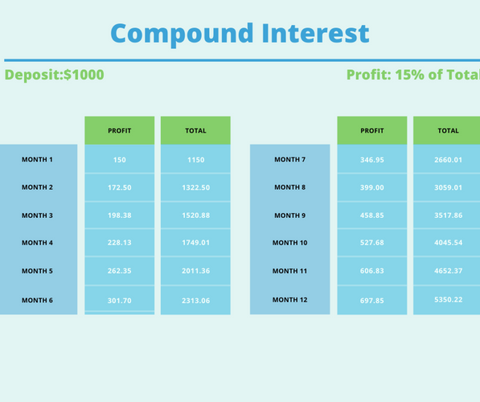demo, finance, stocks -

# Compound Interest: The 8th Wonder of the World

Albert Einstein famously referred to Compound interest as the 8th Wonder of the world. As he said, “The most powerful force in the universe is compound interest and the greatest mathematical discovery of all time”.

Compound interest is so powerful yet the mathematics behind it is very simple. It is the interest earned on top of the principle and interest. For instance, interest accrues not only on the initial principal deposit you invested but will also accumulate interest over time. This develops a snowball effect where your capital rolls down the hill, it gathers more and more interest until you end up with a very large snowball from what you started with. The great thing about compound interest is that it’s available to EVERYONE to utilize. The day you decide to utilize it, is the day you will see the wonders of compound interest. Compound interest is the magic bullet of your savings and investing strategy.Here is the Magic Formula:

The compound interest formula where the Interest is compounded once a year is

A = P(1+R)Y

• A = the accumulated amount i.e. how much money you’ve accumulated after n years, including interest.
• R = the rate of interest (AER) as a decimal (7% means =.07)
• Y = the number of years you leave it on deposit

The two Levels of Compound Interest: Frequency & Time

In the above example, we calculated compounding annually. Some investments may compound quarterly or even monthly. Below are the formulas to calculate quarterly and monthly compound interest.

Quarterly Compounding = P (1+ R/4)4

Monthly Compounding= P(1 + R/12) 12The more frequent the interval of compounding is, the bigger the impact will be on compound growth. Even though frequency is an important lever for your future value of savings, the length of time is just as impactful on your investment growth.

Compound interest exercises its most dramatic effect when the term (Time) is extended for a given interest. This means, the longer an amount is compounding, the greater the effect. For instance, if you invested \$10,000 using the above formula, compounding at 8% per year, over 10 years the future value would be \$12,597. If you take the same principal sum and interest rate, but compound it for over 25 years, the future value would be \$21, 589. The original principal of \$10,000 doubles just with compound interest accumulation.

This is how you can become a millionaire, if you can convert to the habit of saving, and investing then you will come to see that compound interest is your long-term best friend on your journey to create accumulating wealth. Having your money working for you, rather than the other way around is definitely achievable thanks to compound interest. Your coffee change daily can be saved and turned into millions over 20 or 30 years. If you Invested \$5000 a year with a return of 7% from the age of 25, you would be a millionaire by 65. So all you have to do is put aside \$417 a month towards compound interest investing, or rebudgeting your spending habits, and you’ll be on the road to being a millionaire.

To start:

• Saving and/or Investing a regular amount of money each month.
• Leaving you money invested for the long-term.
• Reinvesting your gains (interest), again and again.